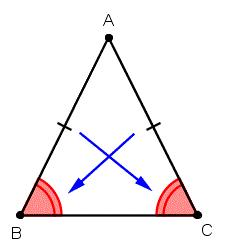# Calculate 4865

Calculate the length of the line segment AB, given A [8; -6] and B [4; 2]

x =  8.9443

### Step-by-step explanation:Did you find an error or inaccuracy? Feel free to write us. Thank you!

Tips for related online calculators
Line slope calculator is helpful for basic calculations in analytic geometry. The coordinates of two points in the plane calculate slope, normal and parametric line equation(s), slope, directional angle, direction vector, the length of the segment, intersections of the coordinate axes, etc.
Do you want to convert length units?
The Pythagorean theorem is the base for the right triangle calculator.